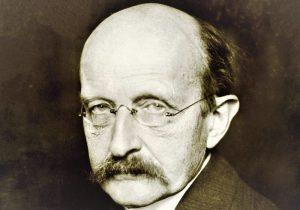Physics

# Planck constant

Planck constant is one of the fundamental constants of physics proposed by Max Planck in 1900 to explain a black body radiation. The irradiated energy cannot take any kind of values but only entire multiple values of a quantum of energy. The Planck constant links the energy value with the radiation frequency: E=hf. Planck's works marked the beginning of quantum physics: light (and all forms of radiation) is emitted, transmitted or absorbed by discrete amounts of energy, the "quanta" of energy.Related topics

Planck time, Quantum mechanics

## What is the Planck constant?

It is a quantum physics constant that allows us to determine the amount of energy corresponding to a quantum when it is used to multiply the frequency of a radiation. It owes its name to the German physicist Max Planck, born in 1858.

## History of the Planck constant

It could be said that Max Planck discovered the constant that carries his surname in 1900 in a forced way, because at that time, it was believed that energy exchanges between matter and radiation were carried out continuously, while experiments proved the opposite.

On the evening of October 7, 1900, Max Planck and his colleague Heinrich Rubens discuss the latter’s measurements around a black body radiation (a black body perfectly absorbs all electromagnetic radiation), one of the most difficult problems of the time. That same night, Planck empirically finds the law that describes the behavior of a black body, observed by Rubens.

Two weeks later, Planck and Rubens present their work at Berlin University. Then, on December 14 of that year, at the German Society of Physics, Planck exposes the hypothesis that led him to this law: the energy quantification. There, he explains that the radiation emitted by a black body behaves as if it were constituted by “packages” of energies whose value would be ε = hν, where he sees the frequency of the radiation and h is the constant known today as the Planck constant.

This is how Planck solves the problem of the black body, raised by the physicist Gustave Kirchhoff in 1859, who had studied the behavior of bodies in thermal equilibrium with the radiation that surrounds them.

Finally, it is worth noting that in 1918, the German physicist received a Nobel prize for his decisive discoveries that opened the way to quantum mechanics. Indeed, Planck’s constant, which associates the energy of a particle with its wavelength, constitutes the fundamental magnitude of quantum mechanics.

## What is the Planck constant for?

The Planck constant is used to describe the quantification phenomena that occur with particles, and from which certain physical properties take only multiple values from fixed values instead of a continuous set of possible values. For example, the energy of a particle is associated with its frequency by:

These quantification conditions are found throughout quantum mechanics.

## Value

The physicist Max Planck made a great contribution to quantum theory by discovering the value of a constant that would bear his name, and that would express the minimum threshold of energy that can be measured in a particle.

The value of this constant is: h = 6.63 . 10 -34 joules per second.

## Planck constant formula

The formula developed by Max Planck is: E=h·f, in which:

• E= energy of the frequency;
• h= Planck constant;
• f= wave frequency.

Later, the physicist gives the name “quantum” to these quantities.

## Importance

Planck constant introduces the discontinuity in the description of elementary phenomena, which constitutes the basis of quantum physics.

This is how the importance of Planck’s discovery does not consist in a formal operation or in his mathematical ability. In reality, the transcendence of his proposal resides above all in the revolutionary interpretation of the physical sense of the constant h.

From the beginning, Planck attributed to the constant the name of “action elemental quantum” because it possesses the dimensions of an action (energy multiplied by a time) and because it only intervenes by multiple wholes. Thus, Planck introduced the idea of a granular composition when all physicists thought that continuity reigned.

To conclude, it can be appreciated that thanks to Planck’s formula the energy of a radiation can be measured, not only in a unit of energy, but also in units of length and frequency. Also, by using the law of the black body, one can determine the temperature of an object whose emission is centered on a certain frequency.

Written by Gabriela Briceño V.